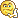#www.vustudents.ning.com

We non-commercial site working hard since 2009 to facilitate learning Read More. We can't keep up without your support. Donate.

 Instructions: Please read the following instructions carefully before submitting assignment. It should be clear that your assignment will not get any credit if:   §         The assignment is submitted after due date. §         The submitted assignment does not open or file is corrupt. §         Assignment is copied(partial or full) from any source (websites, forums, students, etc)   Note: You have to upload only .cpp file. Assignment in any other format (extension) will not be accepted and will be awarded with zero marks. For example, if you submit code in .doc (Word document) or .txt files, no reward will be given in any case.     Objective:   The objective of this assignment is to provide hands on experience of:   §         Objects and Classes §         Constructors and Destructors in classes §         Setters and Getters in classes §         Use of friend functions §         Operator overloading     Guidelines:   §         Code should be properly indented and well commented. §         Follow C/C++ rules while writing variable names, function names etc §         Use only dev-C++ for this assignment. Assignment Problem Statement:     You are required to write a program for calculating area of Trapezoid. Formula for calculating area of trapezoid is   Where a and b are two bases of trapezoid and h corresponds to height.     Detailed Description:   Create a class named Trapezoid which contains two bases and height as data members. Implement a default constructor to initialize all data members with zero and a parameterized constructor which takes three arguments to initialize data members of class. Take input from user for base1, base2 and height of 2 objects. Overload + operator for the class Trapezoid in such a way that corresponding elements of both objects of the same class can be added. Also implement a friend function named calculateArea() which takes two objects of the class Trapezoid as arguments, adds both objects using overloaded + operator and calculates the area of resultant Trapezoid object.           Sample output:

Views: 3822

Attachments:

### Replies to This Discussion

Aliza ap ne error kesay theek kia?CS201 Assignment # 4 ; Due date 28 Jan 2013
Complete Working Solution..

Please change the some variable names...

Attachments:

file compile nahi ho rahi dost

Complete Solution.......

//////////////////////////Assignment NO .4 ///////////////////////////////////

#include <iostream>
#include <stdlib.h>
#include <conio.h>

using namespace std;

class Trapezoid{

public:
Trapezoid();
Trapezoid(float,float,float);
Trapezoid operator +(Trapezoid&);
void GetInput();
void SetBase1(float);
void SetBase2(float);
void SetHeight(float);
void display();
friend void CalculateArea(Trapezoid,Trapezoid);

private:
float base1,base2,height;
};
Trapezoid::Trapezoid()
{
base1 = 0.0;
base2 = 0.0;
height = 0;

}
Trapezoid::Trapezoid(float b1,float b2,float h)
{

base1 = b1;
base2 = b2;
height = h;

}
void Trapezoid::display()
{
coutendl;
cout"After adding both objects by using overloaded + operator\n"endl;
cout"Base 1 = " base1endl;
cout"Base 2 = " base2endl;
cout"Height = " heightendl;
coutendl;
cout"Area of Trapezoid : "(base1+base2)/2*height;
}
void Trapezoid::SetBase1(float b1)
{
base1 = b1;

}
void Trapezoid::SetBase2(float b2)
{
base2 = b2;
}
void Trapezoid::SetHeight(float h)
{
height = h;
}

Trapezoid Trapezoid :: operator + (Trapezoid & trap)
{
Trapezoid temp;
temp.base1 = base1 + trap.base1;
temp.base2 = base2 + trap.base2;
temp.height = height + trap.height;
return temp;

}
void Trapezoid::GetInput()
{
float b1 = 0.0;
float b2 = 0.0;
float h = 0;
coutendl;
cout"Enter Base 1:";
cin>>b1;
cout"Enter Base 2:";
cin>>b2;
cout"Enter Height:";
cin>>h;
SetBase1(b1);
SetBase2(b2);
SetHeight(h);
}
void CalculateArea(Trapezoid b1,Trapezoid b2)
{
Trapezoid temp;
temp = b1 + b2;
temp.display();
}
int main()
{
Trapezoid b1;
Trapezoid b2;
coutendl;
cout"Enter Data for Object 1 :\n";
b1.GetInput();
cout"Enter Data for Object 2 :\n";
b2.GetInput();
CalculateArea(b1,b2);
getch();
}

Attachments:

## CS201 Assignmnt#4 solution

100% ok solution

#include <iostream>
#include <stdlib.h>
#include <conio.h>
#include <stdio.h>
using namespace std;
class trapezoid{
public:
trapezoid();
trapezoid(float,float,float);
trapezoid operator +(trapezoid&);
void getinput();
void set_base1(float);
void set_base2(float);
void set_hight(float);
void display();
friend void calculateArea(trapezoid,trapezoid);

private:
float base1,base2,hight;
};
trapezoid::trapezoid()
{
base1 = 0.0;
base2 = 0.0;
hight = 0;

}
trapezoid::trapezoid(float bs1,float bs2,float ht)
{
base1 = bs1;
base2 = bs2;
hight = ht;

}
void trapezoid::display()
{
cout"base 1: "base1endl;
cout"base 2: "base2endl;
cout"hight : "hightendl;
cout"The Eare of the Trapezoid is: "(base1+base2)/2*hightendl;
}

void trapezoid::set_base1(float bs1)
{
base1 = bs1;

}
void trapezoid::set_base2(float bs2)
{
base2 = bs2;
}
void trapezoid::set_hight(float ht)
{
hight = ht;
}

trapezoid trapezoid::operator+(trapezoid& trap)
{
trapezoid temp;
temp.base1 = base1 + trap.base1;
temp.base2 = base2 + trap.base2;
temp.hight = hight + trap.hight;
return temp;

}
void trapezoid::getinput()
{
float bs1 = 0.0;
float bs2 = 0.0;
float ht = 0;
cout"Please Enter Base 1: ";
cin>>bs1;
cout"Please Enter Base 2: ";
cin>>bs2;
cout"Please Enter Hight: ";
cin>>ht;
set_base1(bs1);
set_base2(bs2);
set_hight(ht);
}
void calculateArea(trapezoid bs1,trapezoid bs2)
{
trapezoid temp;
temp = bs1 + bs2;
temp.display();

}
int main()
{
trapezoid bs1;
trapezoid bs2;
cout"Object {1}"endl;
bs1.getinput();
coutendl;
cout"Object {2}"endl;
bs2.getinput();
calculateArea(bs1,bs2);
_getch();

}

## CS201 Assignmnt#4 solution

100% ok solution

Attachments:

cout"base 1: "base1endl;
is line ma q error aa raha hy..??? :/

koi error ni hai. Thanks tariq bhaiJazaak ALLAH

Hello! Friends, anybody solved the CS201 Assignment 4 at his own?

I would like to understand full assignment step by step, if anybody could do, this will be a great help and i will appreciate that, thx in advance